# Python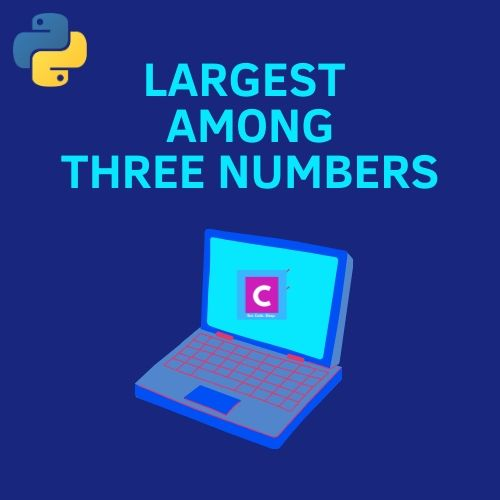## Python 3 Program to Find Largest Among Three Numbers

In this program, you will find the simple Python 3 program to find the largest among the three numbers. To understand this program, you must have basic knowledge of Python programming concepts such as variables, operators, and conditional statements. Here are some recommended Python books for beginners that you can use in your Python journey. …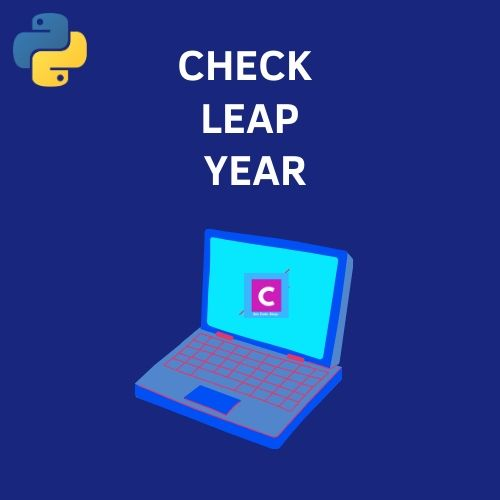## Python 3 Program to Check Leap Year

In this tutorial, you will find a simple Python 3 program to check leap year. To understand this program, you should have a basic understanding of Python variables, operators, and if-else statements. In case, you are just getting started with Python, then I will recommend you to get any of these Python books for beginners. …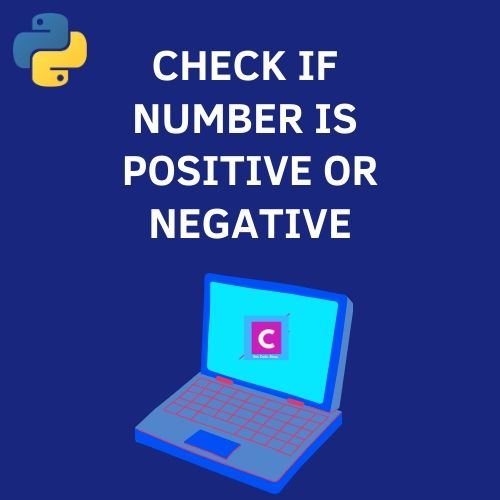## Python 3 Program To Check If Number Is Positive Or Negative

In this tutorial, you will find a simple Python 3 program to check if a number is positive or negative. Before moving ahead, you should know about the basics of Python programming language. If you have just started your Python journey, then do check out these best books for beginners to learn Python. Also Read: How …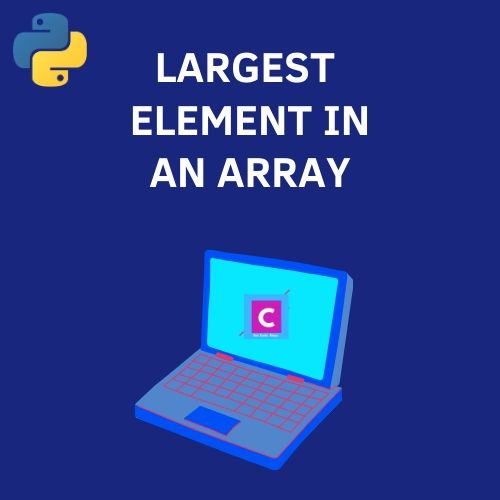## Python 3 Program To Find Largest Element In An Array or List

In this tutorial, you will learn a simple Python 3 program to find the largest element in an array or list using functions. Before proceeding further, you must be aware of the basics of Python programming language, such as how to use variables, create functions, etc. If you have just begun your Python journey, then …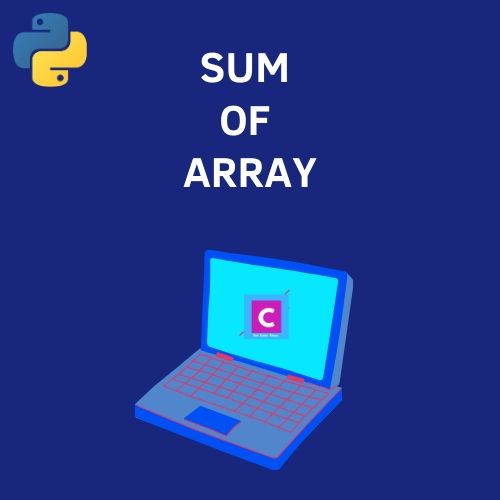## Python 3 Program To Find The Sum of An Array

In this tutorial, you will learn a simple Python program to find the sum of an array. To understand this Python program, you should have an understanding of the following concepts: Python programming language Variables and datatypes Lists Functions We can do this by 2 methods. First, we can calculate the sum of all the …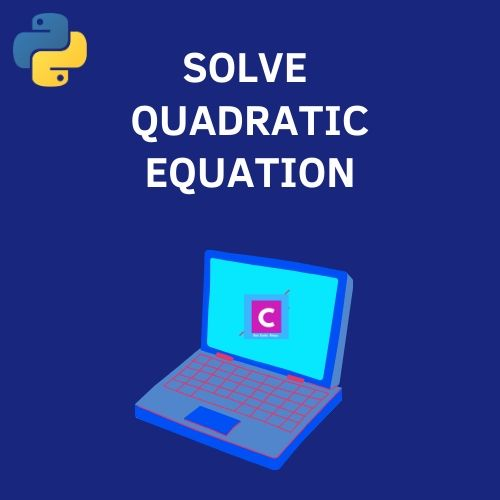## Python 3 Program To Solve A Quadratic Equation

In this tutorial, you will find the Python 3 program to solve a quadratic equation. To understand this example, you should have the basic knowledge of Python programming concepts such as variables, data types, and operators.  If you are looking for some good books to learn Python, then do check out our recommended list.  Also …

Scroll to Top